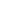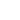# 【原创】循环神经网络入门

``````# 用全局变量存储随机数种子
seed = 0

def random(section):
global seed
seed = next_random(seed)
output = process(seed, section)
return output
``````

``````# 将状态作为输入传递
def random(section, old_seed):
seed = next_random(old_seed)
output = process(seed, section)
# 额外返回状态
return (output, seed)
``````

``````rnn = RNN(D, H)
``````

``````h = rnn.next(h0, x)
``````

``````h = rnn.next(x)
``````

`h`的具体计算方法为：

``````h[t] = tanh(Wh(h[t- 1]) + Wx(x[t]) + b)
``````via colah.github.iovia arunmallya.github.io
``````# LSTM和原生循环网络的总体结构是一致的。
lstm = nn.LSTM(D, H)

# 输入门，决定是否将信息传入细胞
i[t] = sigmoid(ai[t])
# 遗忘门，决定是否遗忘信息
f[t] = sigmoid(af[t])
# 输出门，决定细胞状态中哪些信息将被输出
o[t] = sigmoid(ao[t])
# 使用`tanh`调整输出结果（确保输出值在-1和1之间）
g[t] = tanh(ag[t])

# 细胞状态既取决于上一个时刻的细胞状态，
# 也取决于输入门和遗忘门的作用
c[t] = f[t] * c[t - 1] + i[t] * g[t]
# 状态取决于输出门和细胞状态
h[t] = o[t] * tanh(c[t])
``````

### 评论游客

2017-10-26

2017-11-22

2017-11-09

2018-01-12

### 聚焦3C智造，华南机器人应用市场领跑全国发布需求联系客服